Question

# Find the weighted estimate, p (with a line over it) to test the claim that p...

Find the weighted estimate, p (with a line over it) to test the claim that p 1 = p 2. Use α = 0.05. Assume the samples are random and independent. Sample statistics: n 1 = 50, x 1 = 35, and n 2 = 60, x 2 = 40.

Given that,

From given values ,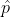= (x1 + x2) / (n1 + n2) = (35 + 40) / (50 + 60) = 75 / 110 = 0.682

the weighted estimate, p = 0.682

#### Earn Coins

Coins can be redeemed for fabulous gifts.

Similar Homework Help Questions
• ### Find the critical value to test the claim that μ1 < μ2. Two samples are random,...

Find the critical value to test the claim that μ1 < μ2. Two samples are random, independent, and come from populations that are normal. The sample statistics are given below. Assume that σ 2/1= σ2/2. Use α = 0.05. n1 = 15 n2 = 15 x1 = 25.74 x2 = 28.29 s1 = 2.9 s2 = 2.8

• ### Find the critical values, t0, to test the claim that μ1 = μ2. Two samples are...

Find the critical values, t0, to test the claim that μ1 = μ2. Two samples are random, independent, and come from populations that are normal. The sample statistics are given below. Assume that σ 2 1 ≠ σ 2 2 . Use α = 0.05. n1 = 32 n2 = 30 x1 = 16 x2 = 14 s1 = 1.5 s2 = 1.9

• ### Answer 13 and 15! Answer 13 and 15 In Exercises 13-16, (a) find the test statistic,...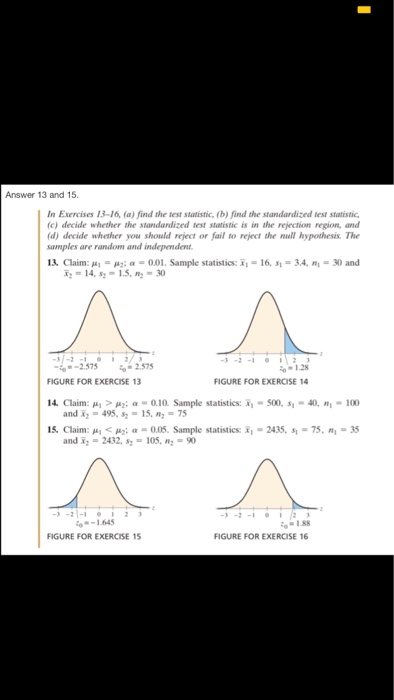Answer 13 and 15! Answer 13 and 15 In Exercises 13-16, (a) find the test statistic, (b) find the standardized test statistic (c) decide whether the standardized test statistic is in the rejection region, and (d) decide whether you should reject or fail to reject the null hypothesis. The samples are random and independent 13, Claim: , .. μα α-0.01. Sample statistics: x". 16, sı 3.4. ni 30 and 14, s-15, -30 37-2 -2.575 to 2.575 01.28 FIGURE FOR EXERCISE...

• ### Test the claim about the difference between the two population means µ1 and µ2 at the...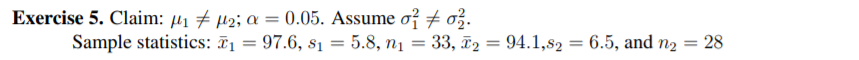Test the claim about the difference between the two population means µ1 and µ2 at the level of significance α. Important! Please remember to include all 5 parts of a hypothesis test mentioned in the module summary. Assume the samples are random and independent, and the populations are normally distributed. Exercise 5. Claim: /142; a = 0.05. Assume o o%. Sample statistics: = 97.6, s1 = 5.8, n1 = 33, T2 = 94.1,82 = 6.5, and n2 = 28 Exercise...

• ### Find the critical values, to to test the claim that μι-u2 Two samples are rando given...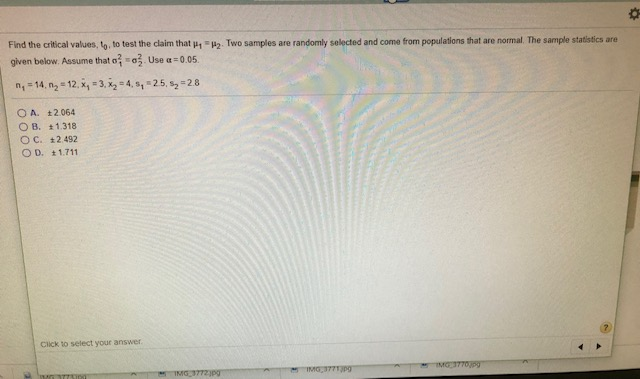Find the critical values, to to test the claim that μι-u2 Two samples are rando given below. Assume that σ' σ, Use α :0.05 y selected and come from populations tat are normal. The sample statistics are 25, s2 28 n, -14, n2 12, x, 3,x24, 1 O A. t2.064 OB. t1.318 O C. +2492 Click to select your answer

• ### Find the standardized test statistic to test the claim that μ1 ≠ μ2. Assume the two...

Find the standardized test statistic to test the claim that μ1 ≠ μ2. Assume the two samples are random and independent. Population statistics: σ1 = 0.76 and σ2 = 0.51 Sample statistics: x1 = 3.6, n1 = 51 and x2 = 4, n2 = 38

• ### Test the indicated claim about the means of two populations. Assume that the two samples are...

Test the indicated claim about the means of two populations. Assume that the two samples are independent simple random samples selected from normally distributed populations. Do not assume that the population standard deviations are equal. Use the traditional method or P-value method as indicated. A researcher was interested in comparing the response times of two different cab companies. Companies A and B were each called at 50 randomly selected times. The calls to company A were made independently of the...

• ### Find the standardized test statistic to test the claim that μ1 < μ2. Two samples are...

Find the standardized test statistic to test the claim that μ1 < μ2. Two samples are random, independent, and come from populations that are normally distributed. The sample statistics are given below. Assume that σ 2 /1 = σ 2 /2 . n1 = 15 n2 = 13 x1 = 27.88 x2 = 30.43 s1 = 2.9 s2 = 2.8

• ### Find the standardized test statistic to test the claim that μ1 ≠ μ2. Two samples are...

Find the standardized test statistic to test the claim that μ1 ≠ μ2. Two samples are random, independent, and come from populations that are normally distributed. The sample statistics are given below. Assume that σ 2 /1 ≠ σ 2 /2 . n1 = 11 n2 = 18 x1 = 6.9 x2 = 7.3 s1 = 0.76 s2 = 0.51

• ### Use a t-test to test the claim about the population mean μ at the given level...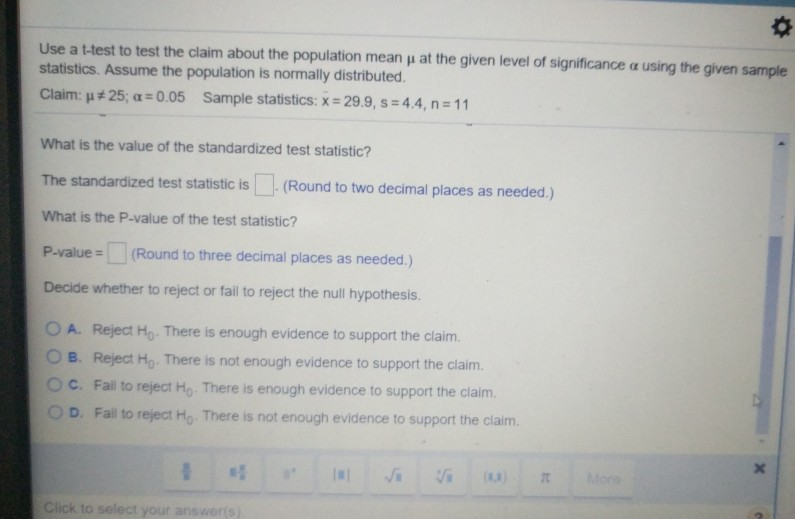Use a t-test to test the claim about the population mean μ at the given level of significance α using the given sample statistics. Assume the population is normally distributed. Claim: μ# 25; α:0.05 Sample statistics: x 29.9, s-44, n 11 What is the value of the standardized test statistic? The standardized test statistic is(Round to two decimal places as needed.) What is the P-value of the test statistic? P-value (Round to three decimal places as needed.) Decide whether to...